# Electronics and Communication Engineering - Measurements and Instrumentation

### Exercise :: Measurements and Instrumentation - Section 1

16.

In figure, Z1 = 200∠60° Ω, Z2 = 400∠ - 90° Ω, Z3 = 300∠0°. Then Z4 for bridge to be balanced is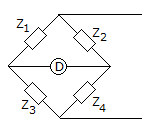A. 150∠30° Ω B. 400∠ - 90° Ω C. 300∠90° Ω D. 600∠ - 150° Ω

Explanation: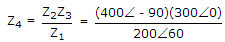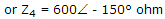.

17.

In a ballistic galvanometer, a charge of 100 μC gives a first swing of 25°. The charge required to cause first swing of 50° is

 A. 200 μC B. 400 μC C. 50 μC D. 25 μC

Explanation:

swing is proportional to charge.

18.

Two resistors R1 = 36 Ω ± 5% and R2 = 75 Ω ± 5% are connected in series. The total resistance is

 A. 111 ± 0 Ω B. 111 ± 2.778 Ω C. 111 ± 5.55 Ω D. 111 ± 7.23 Ω

Explanation: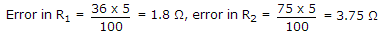Total maximum error ± (1.8 + 3.75) = ± 5.55 Ω.

19.

Figure shows an RC potentiometer to measure ac voltage. It is desired that V0/Vi should be independent of frequency. The value of C should be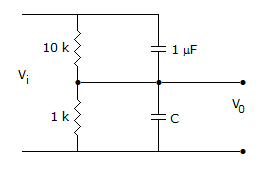A. 10 μF B. 11 μF C. 0.1 μF D. 0.09 μF

Explanation:

Since resistances are in the ratio of 10 : 1, capacitances should be in the ratio of 1 : 10 because XC ∝ 1/C.

20.

In a strain measuring device using a strain gauge, the output quantity is

 A. voltage B. resistance C. impedance D. either (a) or (b)